# Thick_Wall_Cylinder_Variable_E

Model number
0221

This model simulates the pressure and geometry resulting from changes in the internal radius of a thick-walled,

## Description

``` This model simulates the pressure and geometry resulting from changes in
the internal radius of a thick-walled, distensible, isotropic, cylindrical
vessel.  Stresses in the radial and axial directions are neglected and vessel
wall material is assumed to be incompresible.  An external function "ri" sets
the inner radius values within the time domain.  In the available parameter
sets, time in seconds equals the internal radius in centimeters.

The model is used to fit six pressure-radius curves from Drzewiecki et al. 1997 - three
from blood vessels and three from surgical tubes```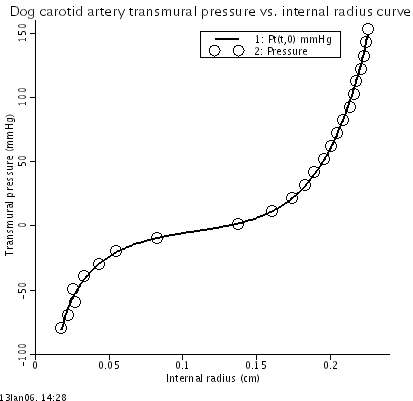## Equations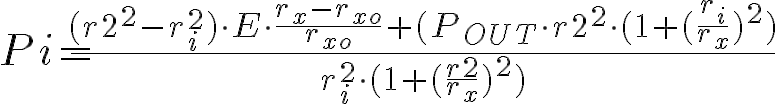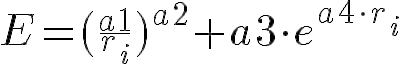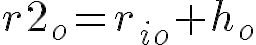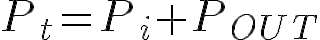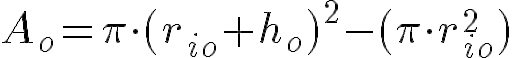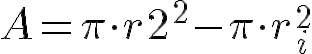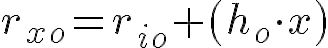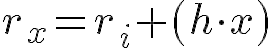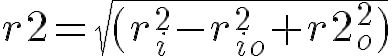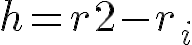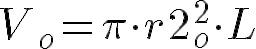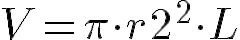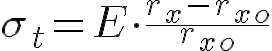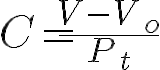The equations for this model may be viewed by running the JSim model applet and clicking on the Source tab at the bottom left of JSim's Run Time graphical user interface. The equations are written in JSim's Mathematical Modeling Language (MML). See the Introduction to MML and the MML Reference Manual. Additional documentation for MML can be found by using the search option at the Physiome home page.

References
```Drzewiecki G, Field S, Moubarak I, and John K.-J. Li: Vessel growth and collapsible
pressure-area relationship. Am J Physiol Heart Circ Physiol 273:H2030-H2043, 1997.

Popov EP.  Mechincs of Materials, SI version.  Prentice Hall.  Englewood Cliffs, NJ. 1978.
```
Key terms
Thick
Variable
Young's modulus
vessel wall
material properties
thick wall